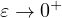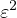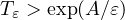Electron. J. Differential Equations, Vol. 2019 (2019), No. 113, pp. 1-21.

### Slow motion for one-dimensional nonlinear damped hyperbolic Allen-Cahn systems Raffaele Folino

Abstract:
We consider a nonlinear damped hyperbolic reaction-diffusion system in a bounded interval of the real line with homogeneous Neumann boundary conditions and we study the metastable dynamics of the solutions. Using an "energy approach" introduced by Bronsard and Kohn  to study slow motion for Allen-Cahn equation and improved by Grant  in the study of Cahn-Morral systems, we improve and extend to the case of systems the results valid for the hyperbolic Allen-Cahn equation (see ). In particular, we study the limiting behavior of the solutions as, whereis the diffusion coefficient, and we prove existence and persistence of metastable states for a time. Such metastable states have a transition layer structure and the transition layers move with exponentially small velocity.

Submitted March 30, 2019. Published October 2, 2019.
Math Subject Classifications: 35L53, 35B25, 35K57.
Key Words: Hyperbolic reaction-diffusion systems; Allen-Cahn equation; metastability; energy estimates.

Show me the PDF file (413 KB), TEX file for this article.Raffaele Folino Dipartimento di Ingegneria e Scienze dell'Informazione e Matematica Università degli Studi dell'Aquila Italy email: raffaele.folino@univaq.it